List of templates

13.1.1. List of templates. Currently the following templates are available:

For rectangular tiles: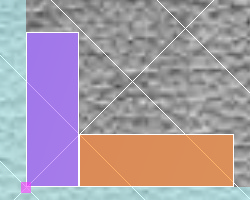With the shift universal

In this template there are two rectangular tiles and, depending on the ratio of their sizes, this layout implements the layout of the type of 'herringbone', 'French layout' or 'chessboard'.

The following templates are added in Version 7.08:Double Herringbone

The rectangular tiles of the same size can be used in the template.

When you select any tile, the entire template is filled with it. Then you can change each element. The size of the tiles must be the same.

The following templates are added in Version 7.10:Offset 1/4, 1/3, 1/2

Rectangular tiles of the same size can be used in this template. When you select any tile, the entire template is filled with it. Then you can change each element. The sizes of the tiles should be the same.

For curled tiles:Octagon with the insert

As an octagon, the following types of tiles can be used: a regular octagon, an irregular octagon and a rectangle with bevels, if the bevels are equal. Square tiles, the sides of which are equal to the size of the bevel of the octagon, are used as the inserts.Fan

The type of tile for this template is a fan.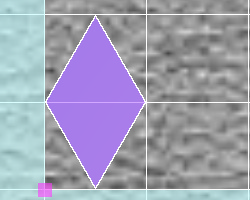Rhombuses  – simple

The type of tile for this template is a simple rhombus.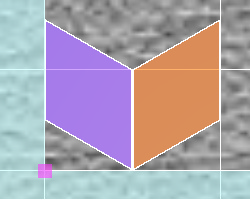Rhombuses – swallow

The type of tile for this template is a simple rhombus. When the first template tile is filled, the second template is filled with the same tile, but further one can be filled with another tile.Rhombuses - honeycombs

The type of tile for this template is a simple rhombus. When the first tile of the template is filled with the same tile, all are filled, but then the templates can be filled with different tiles. There are restrictions on the use of rhombuses in this template - the obtuse angle of the rhombus should be 120 degrees. (ratio of dimensions h = w * v3 or vice versa, where h is the height, w is the width).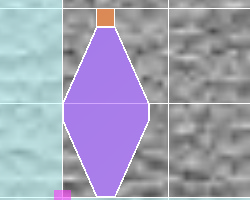Rhombuses with square insert

The type of tile for this template is a rhombus with bevels of corners. Square tiles are used as the inserts, the sides of which are equal to the size of the bevel.Rhombuses with round insert

The type of tile for this template is a rhombus with radius cuts. Round tiles with the rhombus cutout of the radius are used as inserts.Triangles - row

The type of tiles for this template is an isosceles triangle with no radii of curvatures.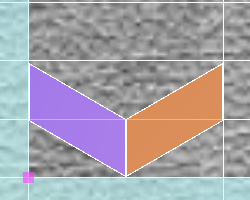Chevrons - swallow_1

The type of tile for this template is the parallelogram left for the first template tile, the parallelogram right for the second one. The sizes of chevrons should be equal.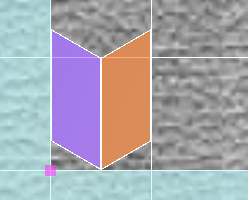Chevrons - swallow_2

The type of tile for this template is the parallelogram right for the first template tile, the parallelogram left for the second one. The sizes of chevrons should be equal.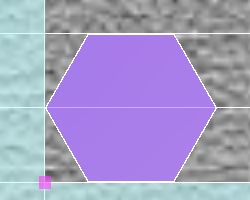Hexagons - honeycombs

The type of tile for this pattern is a regular and irregular hexagon and a rectangle with 2 identical bevels left and right.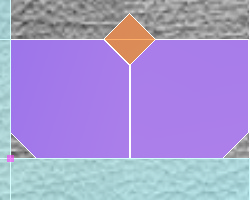Hexagons - with insert

The type of tile for this template is a rectangle with 2 identical bevels left and right. If the tile of the first template is square (h = w), then the second tile of the template is automatically filled (with rotation). Square tiles, the sides of which are equal to the size of the bevel, are used as the inserts.

The following templates were added to Version 7.06: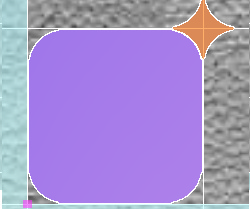Rectangle with rounded corners - with insert

The tile type for this template is a rectangle with rounded corners. As an insert, a rectangle with radius cuts on the sides is used. For the reconciliation of the insert and the base plate should be equal to the radii of recesses, the recess depth of the main tile and shelf size insert, and also the height and width of the main recesses of the tiles should be equal to half the diagonal of the insert.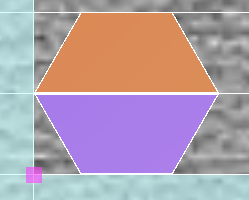Trapezium - Honeycombs

The tile type for this template is a trapezium with a 60-degree angle of inclination of the side faces. When you enter this type of tile in the directory, this parameter is set automatically..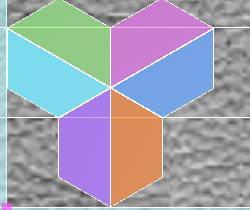Trapezium -  Petal

The tile type for this template is a trapezium with a 60-degree angle of inclination of the side faces. When you select the first tile, the entire template is filled with it. Then you can change each element. The size of the tiles must be the same.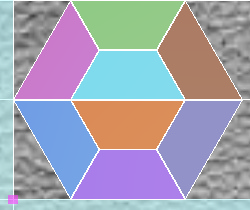Trapezium – Honeycombs_Netting_1

The tile type for this template is a trapezium with a 60-degree angle of inclination of the side faces. When you select the first tile, the entire template is filled with it. Then you can change each element. The size of the tiles must be the same.Trapezium – Honeycombs_Netting_2

The tile type for this template is a trapezium with a 60-degree angle of inclination of the side faces. When you select the first tile, the entire template is filled with it. Then you can change each element. The size of the tiles must be the same.

The following templates were added to Version 7.07: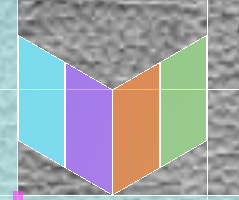Chevrons - Double Swallow

The tile type for this template is the right parallelogram for the first and third tile of the template, and the left parallelogram for the second and fourth tile. The size of the chevrons must be equal.Chevrons - Honeycombs

The tile type for this template is right parallelogram. The angle of inclination of the side is 60 deg. The size of the chevrons must be equal.Chevrons - Herringbone

The tile type for this template is left parallelogram for the first and second tile of the template, right parallelogram for the third and the fourth. The size of the chevrons must be equal. The angle of inclination of the side is 60 deg.

The following templates are added in Version 7.08: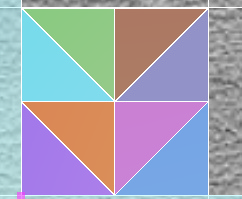Triangles - Arrow

The tile type for this template is rectangular triangles left and right. The triangles sizes of the other two sides must be the same.

When you select any tile, the entire template is filled with it. Then you can change each element. The size of the tiles must be the same.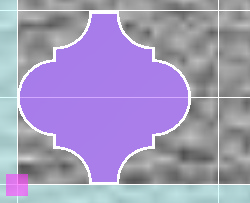Lantern

The tile type for this template is a lantern.

The following templates are added in Version 7.09:Hexagons – Petal

The tile type for this template is a regular hexagon. When you select a tile, the entire template is filled in automatically. Then you can change each element. The size of the tiles must be the same.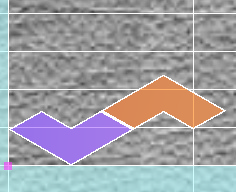Arc – Row_1

The tile type for this template is Arc. When you select a tile, the entire template is filled in automatically. Then you can change each element. The size of the tiles must be the same.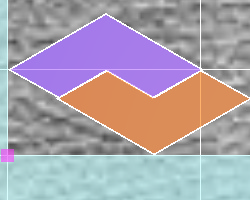Arc – Netting

The tile type for this template is a Arc. When you select a tile, the entire template is filled in automatically. Then you can change each element. The size of the tiles must be the same.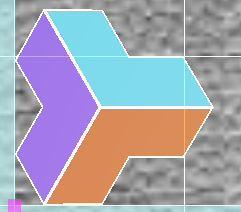Arc – Petal

The tile type for this template is a Arc. When you select a tile, the entire template is filled in automatically. Then you can change each element. The size of the tiles must be the same.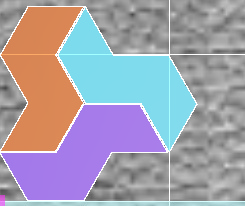Axis – Petal

The tile type for this template is a Axis. When you select a tile, the entire template is filled in automatically. Then you can change each element. The size of the tiles must be the same.

The following templates were added to Version 7.11: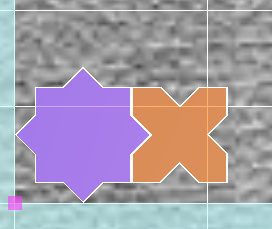Star and Cross

The tile type for this template is a 'Star' and  'CrossForStar'. The sizes of the tiles should be the same.Triangles  -  Row shift 1/2

The tile type for this template is an isosceles triangle with no rounded radii. Each row is shifted by half of the base of the triangle during this laying.

The following templates were added to Version 7.12: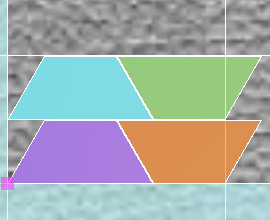Trapezium - Double row without shift

The tile type for this template is a trapezium  with an arbitrary slope of the side faces. When you select a tile, the entire template is filled in automatically. Then you can change each element. The sizes of the tiles should be the same.

The following templates were added to Version 7.13: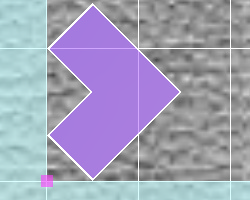Chevrons - Herringbone_Chevron_1

The tile type for this template is Chevron_1 rectangular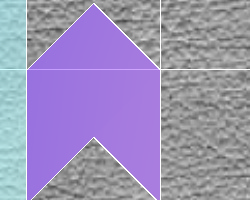Chevrons - Herringbone_Chevron_2

The tile type for this template is Chevron_2 Dovetail

Combined layouts (several types of curled and rectangular tiles):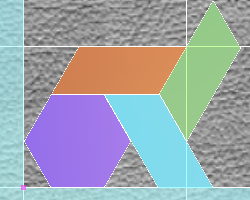Weave_1 (right chevron)

The type of tiles for this template: the first template tile is a regular hexagon (octagon), the rest are right chevrons. Chevrons are filled at the same time with the same tile. The side of the edge of the hexagon should be equal to (approximately) half the base of the chevron.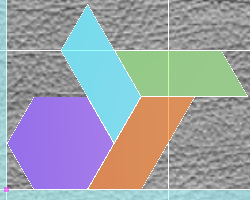Weave_2 (left chevron)

The type of tiles for this template: the first template tile is a regular hexagon (octagon), the rest are left chevrons. Chevrons are filled at the same time with the same tile. The side of the edge of the hexagon should be equal to (approximately) half the base of the chevron.

The following templates were added to Version 7.07: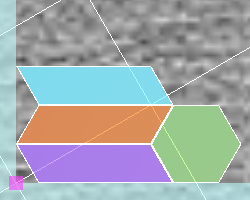Arrow _1

The tile type for this template: the first tile of the template is a left chevron, the second is a right chevron, the third is a left chevron, the fourth is a right hexagon (the octagon). The size of the chevrons must be equal. The angle of inclination of the side isa 60 deg. The side of the hexagon face must be equal to the side face of the chevron.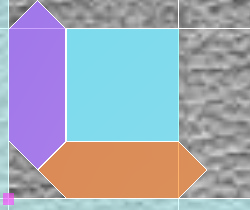Ornament_1

The type of tiles for this template: the first and second tile of the template is an irregular hexagon, the third is a rectangle (square). Hexagons are filled with the same tile at the same time. They must be equal.

The following templates were added to Version 7.13: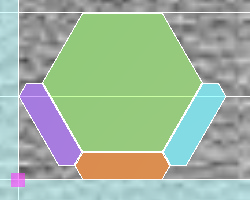Decor_2

The type of tiles for this template: the first three tiles of the template are an irregular hexagon, the fourth is a regular hexagon (octagon). The touching sides of the hexagons must be equal.